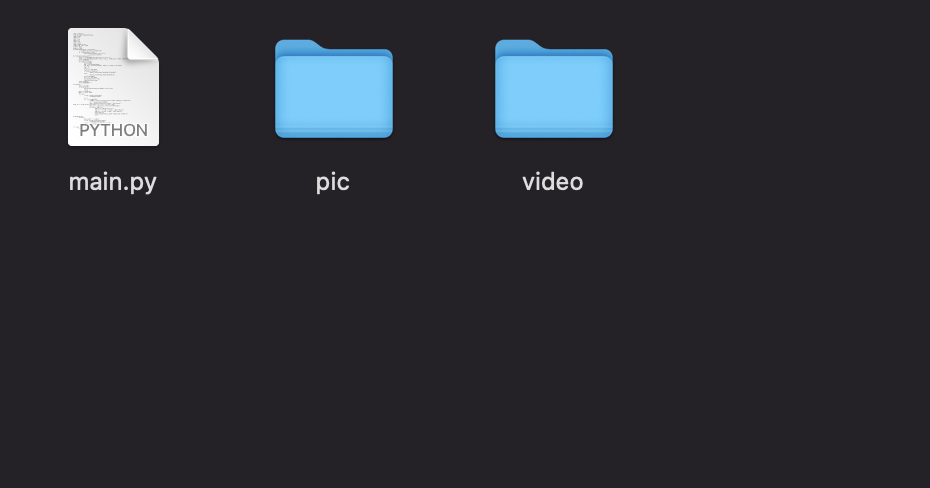# Overview

Do you ever save personality images and character illustrations on your smartphone or computer? Just write the title of the image you want to collect, and we will publish the code that automatically generates the image and the movie to see the image. Please use it. Download a little less than 1000 images from a site called Pre-image.

#We will also include code explanations for those who want to learn scraping.

# code

First of all, I will put the code for those who just want to use it.

#### `main.py`

``````

import requests
from bs4 import BeautifulSoup
import time
import re
import sys
import os
import cv2
import glob
import numpy as np
frame_rate = 0.7  #FPS
width = 1920
height = 1080
r = requests.get(url, stream=True)
if r.status_code == 200:
with open(file_name, 'wb') as f:
f.write(r.content)
def timelaps(images,path):
fourcc = cv2.VideoWriter_fourcc('m','p','4','v')
video = cv2.VideoWriter(path+'.mp4', fourcc, frame_rate, (width, height))
print("During video conversion...")
for image in images:
print(image)
dst_img = np.zeros((height, width, 3), dtype = np.uint8)
top = 0
left = 0
h, w, c = img.shape
if(height/h<width/w):
img=cv2.resize(img,(height*w//h,height))
else:
img=cv2.resize(img,(width,h*width//w))
print(img.shape)
h, w, c = img.shape
dst_img[0:h, 0:w] = img
video.write(dst_img)
video.release()
print("Video conversion completed")
def main():
argv = sys.argv
if(len(argv)<2):
print("python3 main.py \$name\$\Enter n")
return
name = sys.argv
path = './pic/'+name
if(True):
if not os.path.isdir(path):
os.makedirs(path)
c = 0
for i in range(100):
urlName = "https://prcm.jp/list/"+name+"?page={}".format(i+1)
url = requests.get(urlName)
soup = BeautifulSoup(url.content, "html.parser")
#img_list = soup.select('div.entry > ul > li > a > div > img')
img_list = soup.select('div>ul>li>a>div>img')
for img in img_list:
img_url = (img.attrs['src'])
img_url = re.sub('_.*jpeg','.jpeg',img_url)
img_url = re.sub('_.*png','.png',img_url)
print(img_url)
c+=1
# making movie
if(True):
movie_path = './video/'
if not os.path.isdir(movie_path):
os.makedirs(movie_path)
images = sorted(glob.glob(path+"/*.png "))
timelaps(images,movie_path+name)

if __name__ == '__main__':
main()
``````

# Execution method

``````python3 main.py (The name of the image you want)
``````

For example, if you want to collect images of "Kanna Hashimoto"

``````python3 main.py Kanna Hashimoto
``````

When you run itFrom this stateIn this state,The image is saved like this.

# environment

Python3.9.1 Install the required libraries with pip3 or anaconda

# Code description

I will explain the code for those who want to use it on another site or learn the code.

``````import requests
from bs4 import BeautifulSoup
import time
import re
import sys
import os
import cv2
import glob
import numpy as np
frame_rate = 0.7  #FPS
width = 1920
height = 1080
``````

First, import the required library. Enter the FPS, image width (width), and image height (height) here as information on the images to be collected and the video to be created.

``````def download_img(url, file_name):
r = requests.get(url, stream=True)
if r.status_code == 200:
with open(file_name, 'wb') as f:
f.write(r.content)
``````

A function for downloading images. Enter the URL and the name of the file and save the image in the url to the file.

``````def timelaps(images,path):
fourcc = cv2.VideoWriter_fourcc('m','p','4','v')
video = cv2.VideoWriter(path+'.mp4', fourcc, frame_rate, (width, height))
print("During video conversion...")
for image in images:
print(image)
dst_img = np.zeros((height, width, 3), dtype = np.uint8)
top = 0
left = 0
h, w, c = img.shape
if(height/h<width/w):
img=cv2.resize(img,(height*w//h,height))
else:
img=cv2.resize(img,(width,h*width//w))
print(img.shape)
h, w, c = img.shape
dst_img[0:h, 0:w] = img
video.write(dst_img)
video.release()
print("Video conversion completed")
``````

A function that generates a video from the collected images. Create a video by inputting the array of saved image paths and the file name to save the video. It seems that the shape of the saved image does not match the shape of the video I want to make, so I am doing reshape here. However, if you simply use cv2.reshape, the aspect ratio will be messed up, so after expanding to the limit while maintaining the aspect ratio, the rest is filled with black.

``````def main():
argv = sys.argv
if(len(argv)<2):
print("python3 main.py \$name\$\Enter n")
return
name = sys.argv
path = './pic/'+name
if(True):
if not os.path.isdir(path):
os.makedirs(path)
c = 0
for i in range(100):
urlName = "https://prcm.jp/list/"+name+"?page={}".format(i+1)
url = requests.get(urlName)
soup = BeautifulSoup(url.content, "html.parser")
#img_list = soup.select('div.entry > ul > li > a > div > img')
img_list = soup.select('div>ul>li>a>div>img')
for img in img_list:
img_url = (img.attrs['src'])
img_url = re.sub('_.*jpeg','.jpeg',img_url)
img_url = re.sub('_.*png','.png',img_url)
print(img_url)
c+=1
# making movie
if(True):
movie_path = './video/'
if not os.path.isdir(movie_path):
os.makedirs(movie_path)
images = sorted(glob.glob(path+"/*.png "))
timelaps(images,movie_path+name)

if __name__ == '__main__':
main()
``````

It is an execution part. Store the name in sys.argv  in name. Regarding the scraping part, this pre-image site has about 9 image links on the'https://prcm.jp/list/{name}/page = {number}' link, so select it with soup.select. And download each one. The html information can be easily analyzed by selecting Developer and Developer Tool from the View tab of Chrome. If you want to use it on other sites, you need to rewrite this part well. Find out where the image links are buried, select them well with soup.select and add them to the img_list.